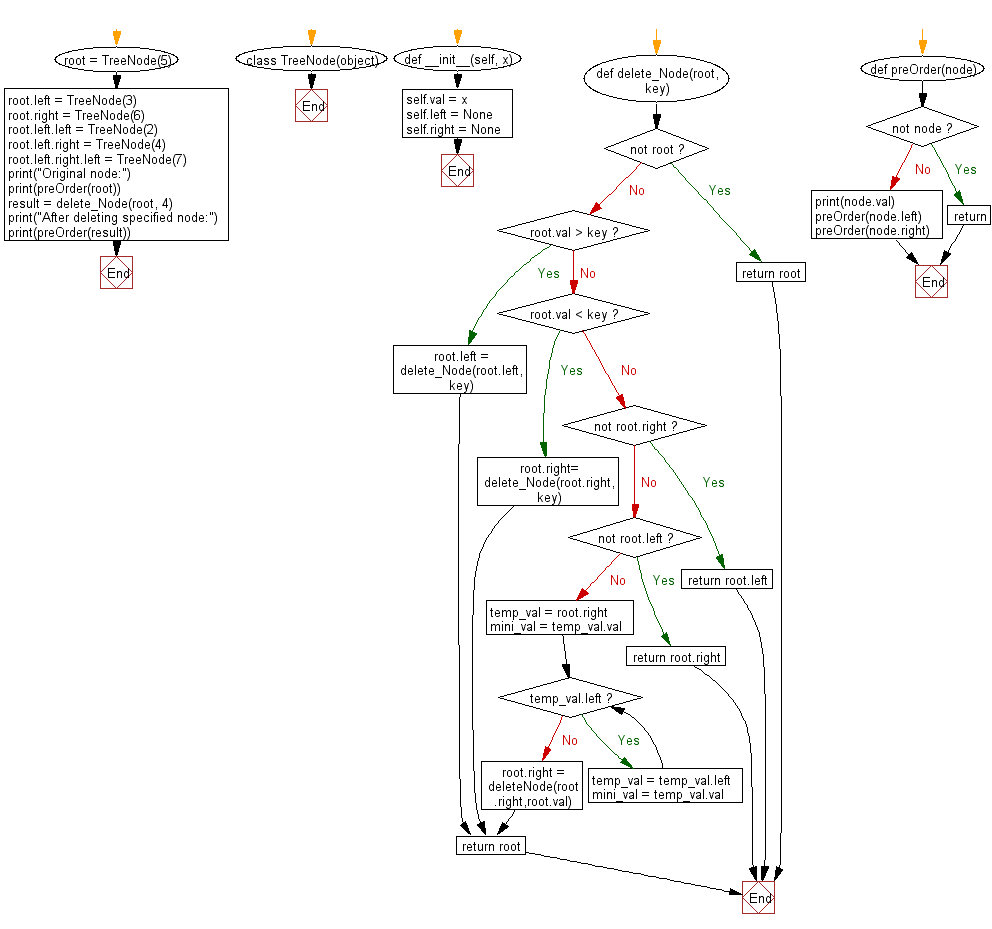﻿ Python Binary Search Tree: Delete a node in a given Binary search tree (BST) - w3resource# Python Binary Search Tree: Delete a node in a given Binary search tree (BST)

## Python Binary Search Tree: Exercise-4 with Solution

Write a Python program to delete a node with the given key in a given Binary search tree (BST).

Note: Search for a node to remove. If the node is found, delete the node.

Sample Solution:

Python Code:

``````# Definition: Binary tree node.
class TreeNode(object):
def __init__(self, x):
self.val = x
self.left = None
self.right = None

def delete_Node(root, key):
# if root doesn't exist, just return it
if not root:
return root
# Find the node in the left subtree	if key value is less than root value
if root.val > key:
root.left = delete_Node(root.left, key)
# Find the node in right subtree if key value is greater than root value,
elif root.val < key:
root.right= delete_Node(root.right, key)
# Delete the node if root.value == key
else:
# If there is no right children delete the node and new root would be root.left
if not root.right:
return root.left
# If there is no left children delete the node and new root would be root.right
if not root.left:
return root.right
# If both left and right children exist in the node replace its value with
# the minmimum value in the right subtree. Now delete that minimum node
# in the right subtree
temp_val = root.right
mini_val = temp_val.val
while temp_val.left:
temp_val = temp_val.left
mini_val = temp_val.val
# Delete the minimum node in right subtree
root.right = deleteNode(root.right,root.val)
return root

def preOrder(node):
if not node:
return
print(node.val)
preOrder(node.left)
preOrder(node.right)

root = TreeNode(5)
root.left = TreeNode(3)
root.right = TreeNode(6)
root.left.left = TreeNode(2)
root.left.right = TreeNode(4)
root.left.right.left = TreeNode(7)
print("Original node:")
print(preOrder(root))
result = delete_Node(root, 4)
print("After deleting specified node:")
print(preOrder(result))
```
```

Sample Output:

```Original node:
5
3
2
4
7
6
None
After deleting specified node:
5
3
2
7
6
None
```

Flowchart:Python Code Editor:

What is the difficulty level of this exercise?

Test your Python skills with w3resource's quiz

﻿

## Python: Tips of the Day

Python: Use Enumerate() In for Loops

```>>> students = ('John', 'Mary',  'Mike')
>>> for i, student in enumerate(students):
...     print(f'Iteration:  {i}, Student: {student}')
...
Iteration: 0, Student: John
Iteration: 1, Student: Mary
Iteration: 2, Student: Mike
>>> for i, student in enumerate(students,  35001):
...      print(f'Student Name: {student}, Student ID #: {i}')
...
Student Name: John, Student ID #: 35001
Student Name: Mary, Student ID #: 35002
Student Name: Mike, Student ID #: 35003
```